NCERT Solutions for Class 10 Maths Chapter 14 Statistics Ex 14.4 are part of NCERT Solutions for Class 10 Maths. Here we have given NCERT Solutions for Class 10 Maths Chapter 14 Statistics Ex 14.4.

 Board CBSE Textbook NCERT Class Class 10 Subject Maths Chapter Chapter 14 Chapter Name Statistics Exercise Ex 14.4 Number of Questions Solved 3 Category NCERT Solutions

## NCERT Solutions for Class 10 Maths Chapter 14 Statistics Ex 14.4

Question 1.
The following distribution gives the daily income of 50 workers of a factory.Convert the distribution above to a less than type cumulative frequency distribution, and draw its ogive.
Solution: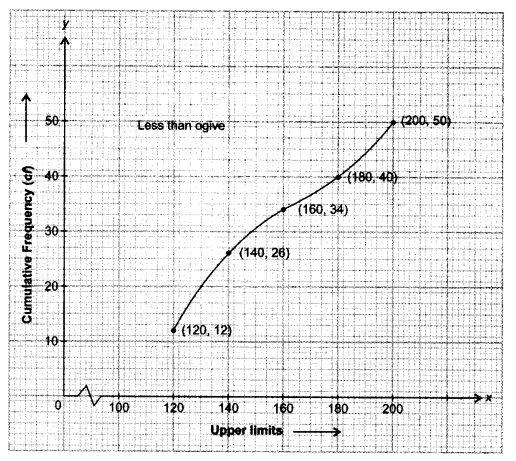Question 2.
During the medical check-up of 35 students of a class, their weights were recorded as follows:Draw a less than type ogive for the given data. Hence obtain the median weight from the graph and verify the result by using the formula.
Solution: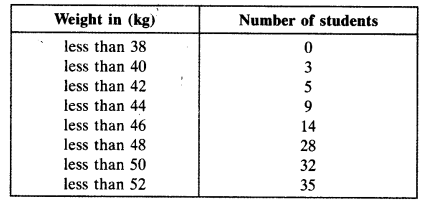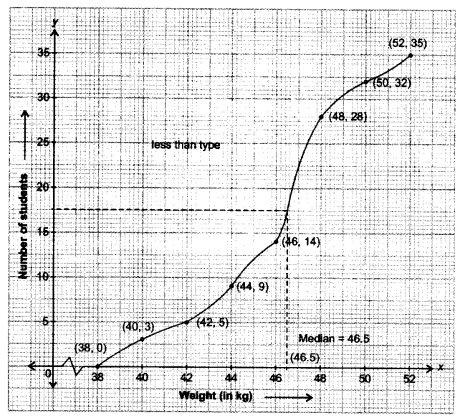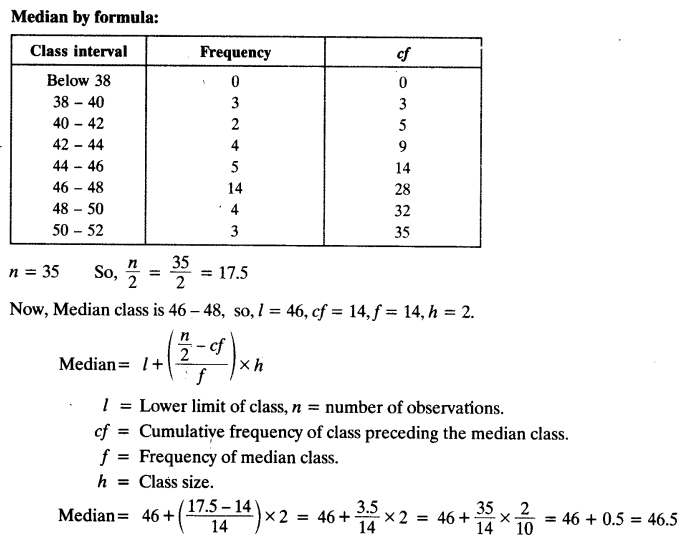Question 3.
The following table gives production yield per hectare of wheat of 100 farms of a village.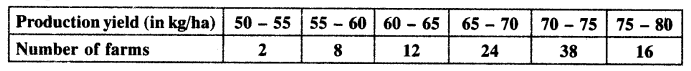Change the distribution to a more than type distribution, and draw its ogive.
Solution: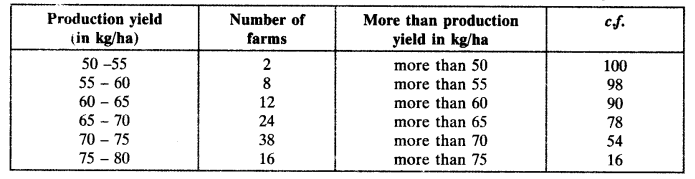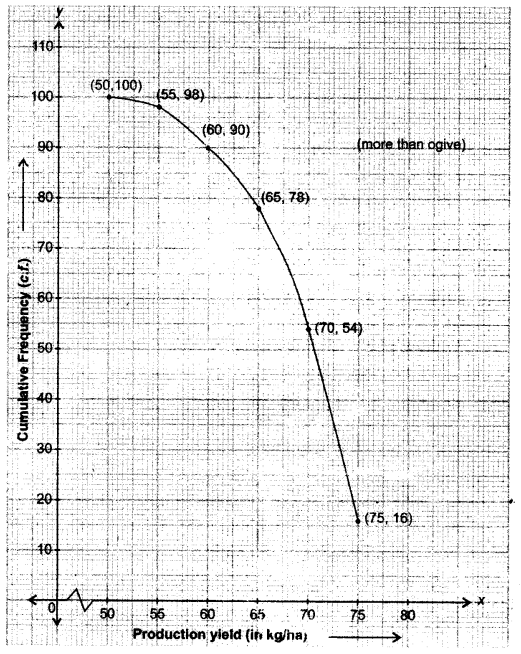We hope the NCERT Solutions for Class 10 Maths Chapter 14 Statistics Ex 14.4, help you. If you have any query regarding NCERT Solutions for Class 10 Maths Chapter 14 Statistics Ex 14.4, drop a comment below and we will get back to you at the earliest.